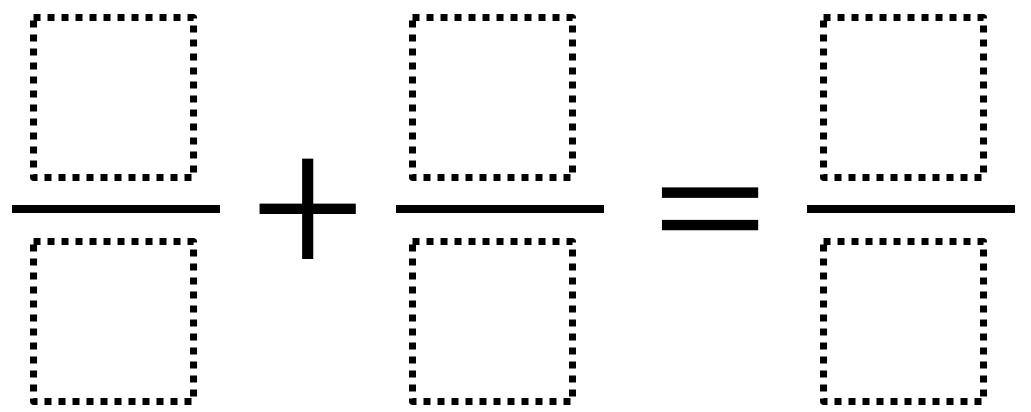Directions: Use the digits 1 to 9, at most one time each, to make a true statement.### Hint

What digits would be challenging as denominators?
What digits would be challenging as numerators?

There are many answers. Here are two possibilities:
2/8 + 1/4 = 3/6
1/2 + 3/9 = 5/6

Source: Owen Kaplinsky

## Equivalent Expressions with Fractions

Directions: Using the digits 0 to 9 at most one time each and choosing either …

1.Another two answers discovered by my students: 1/2 + 9/6 = 8/4 and 5/3 + 2/6 = 8/4

2.2/4 + 1/3 = 12/10 simply it and get 5/6

3.6/8 + 1/2 = 5/4

4.9/3 + 4/2 = 5/1

5.2/1 + 6/9 = 8/3
7/2 + 3/6 = 4/1
8/2 + 9/3 = 7/1

6.8/4 + 6/2 = 5/1
8/4 + 9/3 = 5/1
4/2 + 6/2 = 5/1